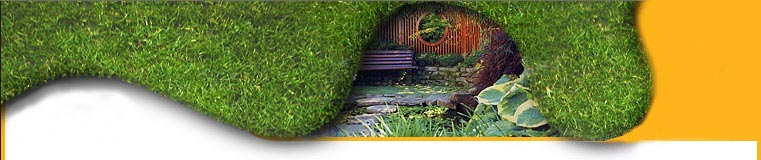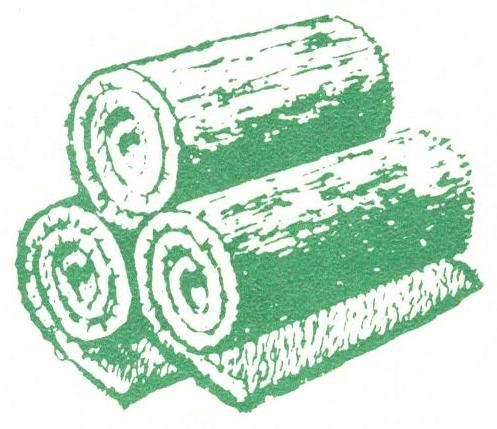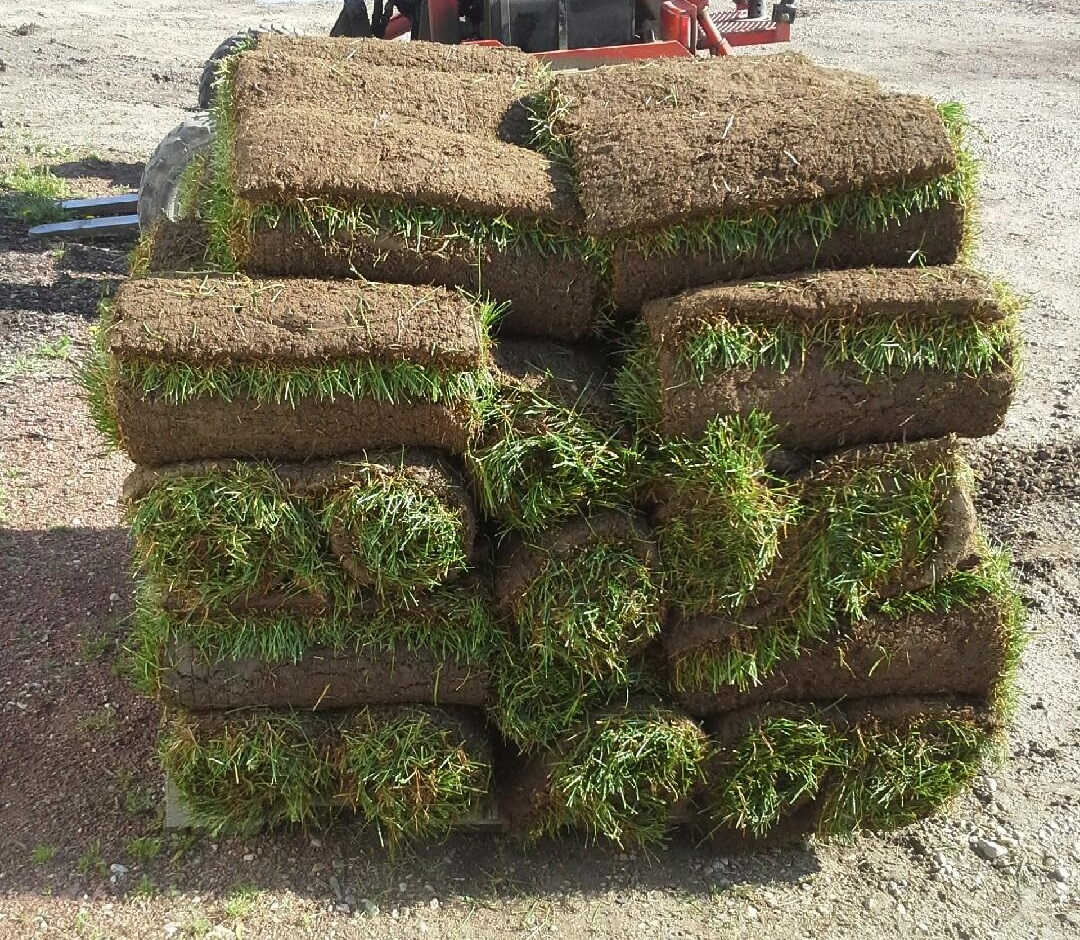Order InfoWe Harvest Fresh Sod  Monday - Saturday  by Appt.

Call for a free estimate, or follow the instructions below to determine the quantity you will need.HomeOrder InfoFAQAbout usContact UsRandom Lake, WI
920-994-4041

How to Measure for Sod

1. First, you need to find the square footage of the area to be sodded.  Below is the equation for finding the square footage.
Square footage of an area = Length x Width

For example, if your front lawn is 80ft long, and 45ft wide, the total area is 3,600 sq ft (80 x 45 = 3,600)

2. Second, now you can convert square feet into square yards. Since there are 9 square feet in a yard, simply divide your area by 9.

For example, if the area of your front lawn is 3,600sq feet, divide by 9 to get 400sq yds (3,600/9 = 400)

Still have questions? Send us an email or give us a call. We’ll do all the calculations for you.

920-994-4041

staff@kriersod.com

Serving the counties of: Milwaukee, Ozaukee, Washington, Waukesha, Sheboygan, Fond du Lac, Manitowoc
Since 1960